Previous: 8.4 Discretization Up: 8.4 Discretization Next: 8.4.2 Drift Term

## 8.4.1 Free Term

In the stationary case without scattering Equation 8.1 degenerates for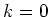as the Wigner potential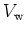is zero for. If also the potential is zero, i.e., the free case, then left (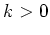) and right (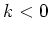) going modes do not couple at all. Accordingly, the solution might become discontinuous for. Discontinuity of the Wigner function foris considered as a feature of ballistic transport and is unavoidable under certain types of inflow boundary conditions. A possibility to get an equation foris to divide Equation 8.1 by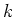and to consider the limit equation for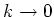.

From meshing constraints discussed in the previous section it follows that the number of grid points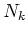is an even number. Hence we can choose the equidistant mesh symmetric aroundexcluding the point.

We implemented four different discretizations for the derivative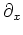: upwinding first and second order and central differences (second and fourth order), see [Bie97]. Special care has to be taken of the boundary points and the points next to the boundary. The discretization at the boundary must be at least exact of order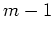if the internal discretization is to be exact of order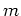. Otherwise the overall order will decrease and be less than.

The discrete formula for the calculation of the current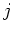is fixed by the discretization of the free streaming operator. If this is not respected then the calculated current density will not be constant in one dimension and conservation of mass is severely violated. This is further discussed in Section 10.1.